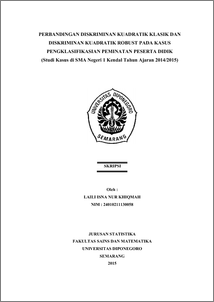PERBANDINGAN DISKRIMINAN KUADRATIK KLASIK DAN DISKRIMINAN KUADRATIK ROBUST PADA KASUS PENGKLASIFIKASIAN PEMINATAN PESERTA DIDIK (Studi Kasus di SMA Negeri 1 Kendal Tahun Ajaran 2014/2015)

KHIQMAH, LAILI ISNA NUR (2015) PERBANDINGAN DISKRIMINAN KUADRATIK KLASIK DAN DISKRIMINAN KUADRATIK ROBUST PADA KASUS PENGKLASIFIKASIAN PEMINATAN PESERTA DIDIK (Studi Kasus di SMA Negeri 1 Kendal Tahun Ajaran 2014/2015). Undergraduate thesis, FSM Universitas Diponegoro.Preview
PDF
596Kb

Abstract

Discriminant is a multivariate statistical technique that can be used to perform the classification new observation into a particular group. Quadratic discriminant analysis tied to an assumption of normal multivariate distributed observations and variance covariance matrix inequality. Robust quadratic discriminant analysis can be used if the observations contain outliers. Classification using robust quadratic discriminant analysis with the Minimum Covariance Determinant (MCD) estimator in the data specialization students of SMA Negeri 1 Kendal that containing outliers gives the results of the classification accuracy of 95,06% with a percentage of 4,94% classification error while generating the classical quadratic discriminant analysis classification accuracy of 92,59% with a percentage of 7,41% classification error. Thus a robust quadratic discriminant analysis with the MCD estimator is more appropriate in the case of the data which contains outliers. Keywords : discriminant, outliers, robust, MCD estimators, classification.

Item Type: Thesis (Undergraduate) H Social Sciences > HA Statistics Faculty of Science and Mathematics > Department of Statistics 47161 Mr Hasbi Yasin 22 Dec 2015 14:42 22 Dec 2015 14:42

Repository Staff Only: item control page